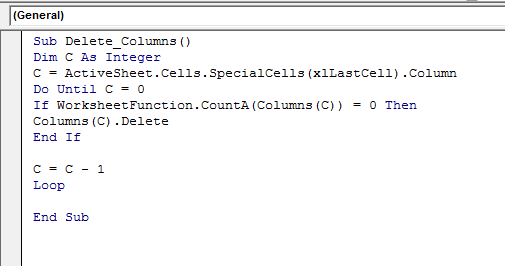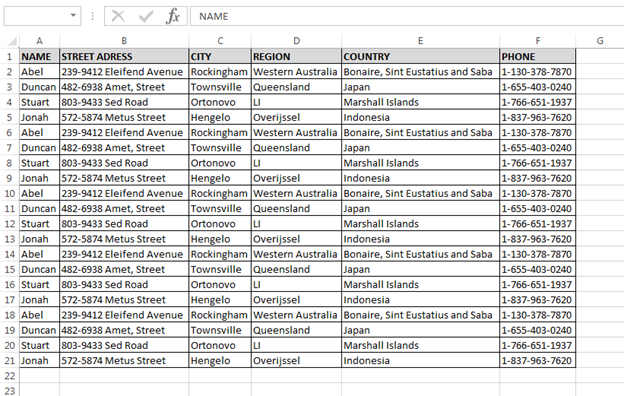How to Delete Empty Columns through VBA in Microsoft Excel?

In this article, we will learn about how to delete empty columns through VBA in Microsoft Excel. We will delete the columns which are completely empty, it means if there is no data within the entire column that data.

Let’s take an example and understand:

We have data in range A1:I21, in which column A contains Name, column B is blank, column C contains Street address, column D City, column E is blank, column F contains region, column G is blank, column H contains country name, and column I contains phone number.We want to delete the blank columns from the data, follow below given steps and code:

• Open the VBE page to press the key Alt+F11
• Insert the module to write the code
• Write the below mentioned code:

Sub Delete_Columns()

Dim C As Integer

C = ActiveSheet.Cells.SpecialCells(xlLastCell).Column

Do Until C = 0

If WorksheetFunction.CountA(Columns(C)) = 0 Then

Columns(C).Delete

End If

C = C - 1

Loop

End Sub

Code Explanation:

First we have defined all the variables. Then the range of blank columns, then we have used “Do until loop” to delete the blank columns. And, now we have defined the blank column and delete command.To run the code press the key F5 on your keyboard,

• All blank columns will get delete.In this way, we can delete the empty columns through VBA in Microsoft Excel.We would love to hear from you, do let us know how we can improve, complement or innovate our work and make it better for you. Write us at info@exceltip.com

1.Here is how to use it to remove if a header is populated but the column is blank below it.

Sub Delete_Columns()

Dim C As Integer

C = ActiveSheet.Cells.SpecialCells(xlLastCell).Column

Do Until C = 0

If WorksheetFunction.CountA(Columns(C)) = 1 Or WorksheetFunction.CountA(Columns(C)) = 0 Then

Columns(C).Delete

End If

C = C - 1

Loop

End Sub

2.Works great for rows too:

Sub Delete_Rows()

Dim R As Integer

R = ActiveSheet.Cells.SpecialCells(xlLastCell).Row

Do Until R = 0

If WorksheetFunction.CountA(Rows(R)) = 0 Then

Rows(R).Delete

End If

R = R - 1

Loop

End Sub

3.How about a version of this where it deletes all blank columns even if there is header text in the header row (and, obviously, would delete that column's header text cell as well...)?

4.Hello-How can you run this exact macro but take into consideration that there may be a column heading? For instance, let's say there is a column heading for Phone number, but no phone numbers are provided-can we run a code to delete empty columns AFTER skipping over the column heading?
Thanks!

5.